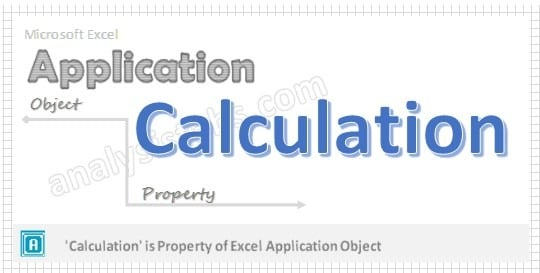Calculation Application Property in VBA represents the calculation mode and returns XlCalculation value. Please find more details about XlCalculation Enumeration values at the end of the chapter. Please find more information about Calculation property in the following chapter.## VBA Calculation Application Property – Syntax

Here syntax for Calculation Property of application object in VBA.

Application. Calculation

Where Calculation as XlCalculation. Please find more details about XlCalculation Enumeration values in the end of the chapter.
In the above syntax Application represents object and Calculation is the Property of Application object.

## VBA Calculation Application Property: Example 1

Please find the below example for Calculation Property of application object in excel VBA. When XlCalculation value is xlCalculationAutomatic, It Controls the Excel recalculation.

```
Sub Window_xlCalculationAutomatic()
Application.Calculation = xlCalculationAutomatic
End Sub
```

## VBA Calculation Application Property: Example 2

Please find the below example for Calculation Property of application object in excel VBA. When XlCalculation value is xlCalculationManual, the user requests for the calculation.

```Sub Window_xlCalculationManual()
Application.Calculation = xlCalculationManual
End Sub
```

## VBA Calculation Application Property: Example 3

Please find the below example for Calculation Property of application object in excel VBA. When XlCalculation value is xlCalculationSemiautomatic, It Controls the Excel recalculation but ignores the changes in tables.

```Sub Window_xlCalculationSemiautomatic()
Application.Calculation = xlCalculationSemiautomatic
End Sub
```

## VBA Calculation Application Property – Instructions

Please follow the below steps to execute the VBA code to save the excel file.
Step 1: Open any existing Excel Application.
Step 2: Press Alt+F11 – This will open the VBA Editor Window.
Step 3: Insert a code module from then insert menu.
Step 4: Copy the above code and paste in the code module which have inserted in the above step.
Step 5: Now press F5 to execute the code.
Step 6: Run the above examples in debug (F8) mode for better understand.

## VBA XlCalculation Enumaration Values

Please find the below VBA XlCalculation Enumaration Values, Name and their description.

Excel Name Value Description
xlCalculationAutomatic -4105 Controls Excel recalculation
xlCalculationManual -4135 User requests for the calculation
xlCalculationSemiautomatic 2 Controls the Excel recalculation but ignores the changes in tables
120+ Professional Project Management Templates!

A Powerful & Multi-purpose Templates for project management. Now seamlessly manage your projects, tasks, meetings, presentations, teams, customers, stakeholders and time. This page describes all the amazing new features and options that come with our premium templates.

LIMITED TIME OFFER
ON SALE85% OFF

Excel Templates

120+ Project Management Templates Pack

Excel | PowerPoint | Word

ULTIMATE RESOURCE MANAGEMENT TEMPLATE

Excel Template

50+ Essential Project Management Templates

Excel | PowerPoint | Word

Project Portfolio Management Templates

Excel | PowerPoint Templates

50+ Excel Project Management Templates

Excel Templates